# Free Body Diagrams, Tutorials with Examples and Explanations

## What is a Free Body Diagram?

The free body diagram helps you understand and solve static and dynamic problem involving forces. It is a diagram including all forces acting on a given object without the other object in the system. You need to first understand all the forces acting on the object and then represent these force by arrows in the direction of the force to be drawn.

## Examples of Free Body Diagrams with Detailed Explanations

### Example 1 : A book on a table

In this example, there are two forces acting on a book at rest on a table:
1) The weight W exerted by the earth on the book
2) The normal force N exerted by the table on the book.### Example 2 : A suspended block

In this example, there are two forces acting on the suspended block at rest:
1) The weight W exerted by the earth on the block
2) The
tension force T exerted by the string (or rope) on the block.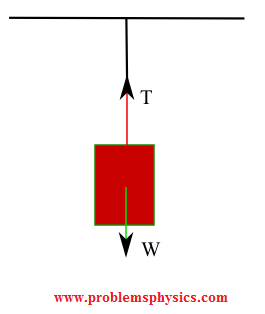### Example 3 : A block on a floor with an acting force Fa to pull the block

In this example, the block is being pulled and therefore a force of friction acts on the block. So there are four forces acting on the block.
1) The weight W exerted by the earth on the block
2) The normal force N exerted by floor on the block.
3) The acting force Fa to pull the block.
4) The
force of friction Ff exerted by floor on the block in the direction opposite the motion due to Fa.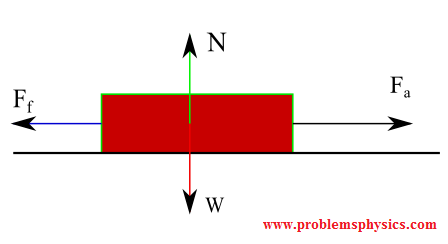### Example 4 : A falling object

In this example, there is only one force action on the falling object.
1) The weight W exerted by the earth on the falling object.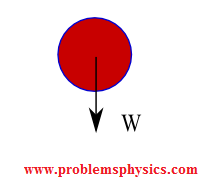### Example 5 : An box on an incline plane (with no frictions)

In this example, we assume that the inclined plane is frictionless; two forces act on the box:
1) The weight W exerted by the earth on the box.
2) The normal force N exerted by the inclined plane on the box. N is normal to the inclined plane.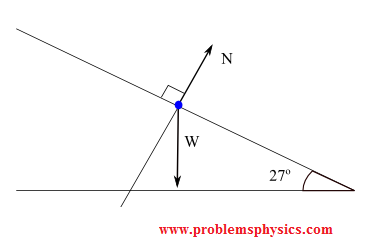### Example 6 : An box on an incline plane with an acting force and friction considered

In this example, a force Fa pulls the box upward and frictions are not negligible. Four forces act on the box:
1) The weight W exerted by the earth on the box.
2) The normal force N exerted by the inclined plane on the box. N is normal to the inclined plane.
3) The acting force Fa.
4) The force of friction F
s exerted by the inclined plane on the box in the direction opposite the motion due to Fa.### Example 7 : A block suspended to the ceiling using three stringsA) free body diagram for the block; two forces (lower part of figure below)
1) The weight W exerted by the earth on the box.
2) The tension force T '
3 exerted by the string on the block.
B) free body diagram of point P; three forces (upper part of figure below)
1) Tension T
1
2) Tension T
2
3) Tension T
3### Example 8 : A system with two blocks, an inclined plane and a pulley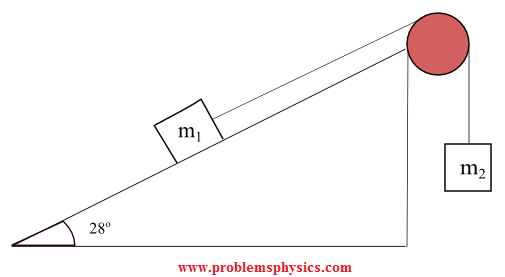A) free body diagram for block m1 (left of figure below)
1) The weight W
1 exerted by the earth on the box.
2) The normal force N
3) The force of friction F
k
4) The tension force T exerted by the string on the block m
1.
B) free body diagram of block m2 (right of figure below)
1) The weight of the block W
2
2) Tension T '.